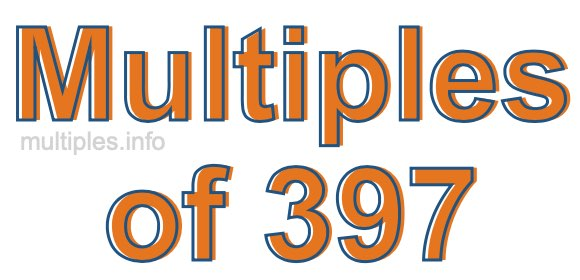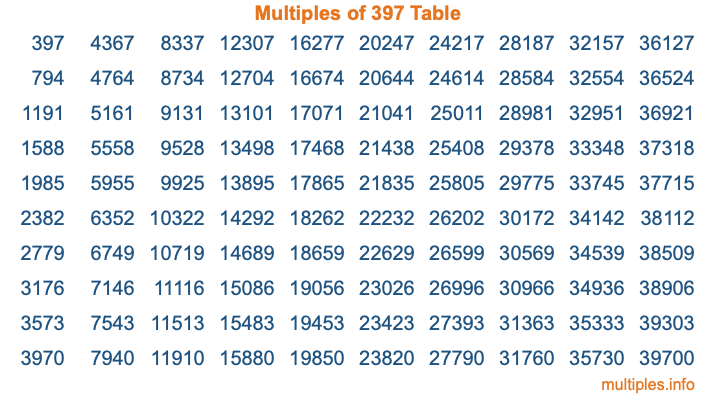Multiples of 397Welcome to the Multiples of 397 page. Here we will first teach you everything you will ever need to know about the multiples of 397, and then give you a study guide summary of everything we taught you to make sure you remember it all. Use this page to look up facts and learn information about the multiples of 397. This page will make you a multiples of three hundred ninety-seven expert!

Definition of Multiples of 397
Multiples of 397 are all the numbers that when divided by 397 equal an integer. Each of the multiples of 397 are called a multiple. A multiple of 397 is created by multiplying 397 by an integer.

Therefore, to create a list of multiples of 397, you start with 1 multiplied by 397, then 2 multiplied by 397, then 3 multiplied by 397, and so on for as long as you want. Thus, the list of the first five multiples of 397 is 397, 794, 1191, 1588, and 1985. To see a larger list of multiples of 397, see the printable image of Multiples of 397 further down on this page. We also have a category where you can choose any nth multiple of 397.

Multiples of 397 Checker
The Multiples of 397 Checker below checks to see if any number of your choice is a multiple of 397. In other words, it checks to see if there is any number (integer) that when multiplied by 397 will equal your number. To do that, we divide your number by 397. If the the quotient is an integer, then your number is a multiple of 397.

Is  a multiple of 397?

Least Common Multiple of 397 and ...
A Least Common Multiple (LCM) is the lowest multiple that two or more numbers have in common. This is also called the smallest common multiple or lowest common multiple and is useful to know when you are adding our subtracting fractions. Enter one or more numbers below (397 is already entered) to find the LCM.

Check out our LCM Calculator if you need more details about the Least Common Multiple or if you need the LCM for different numbers for adding and subtraction fractions.

nth Multiple of 397
As we stated above, 397 is the first multiple of 397, 794 is the second multiple of 397, 1191 is the third multiple of 397, and so on. Enter a number below to find the nth multiple of 397.

th multiple of 397

Multiples of 397 vs Factors of 397
397 is a multiple of 397 and a factor of 397, but that is where the similarities end. All postive multiples of 397 are 397 or greater than 397. All positive factors of 397 are 397 or less than 397.

Below is the beginning list of multiples of 397 and the factors of 397 so you can compare:

Multiples of 397: 397, 794, 1191, 1588, 1985, etc.

Factors of 397: 1, 397

As you can see, the multiples of 397 are all the numbers that you can divide by 397 to get a whole number. The factors of 397, on the other hand, are all the whole numbers that you can multiply by another whole number to get 397.

It's also interesting to note that if a number (x) is a factor of 397, then 397 will also be a multiple of that number (x).

Multiples of 397 vs Divisors of 397
The divisors of 397 are all the integers that 397 can be divided by evenly. Below is a list of the divisors of 397.

Divisors of 397: 1, 397

The interesting thing to note here is that if you take any multiple of 397 and divide it by a divisor of 397, you will see that the quotient is an integer.

Multiples of 397 Table
Below is an image of the first 100 multiples of 397 in a table. The table is in chronological order, column by column. The first column has the first ten multiples of 397, the second column has the next ten multiples of 397, and so on.The Multiples of 397 Table is also referred to as the 397 Times Table or Times Table of 397. You are welcome to print out our table for your studies.

Negative Multiples of 397
Although not often discussed or needed in math, it is worth mentioning that you can make a list of negative multiples of 397 by multiplying 397 by -1, then by -2, then by -3, and so on, to get the following list of negative multiples of 397:

-397, -794, -1191, -1588, -1985, etc.

Multiples of 397 Summary
Below is a summary of important Multiples of 397 facts that we have discussed on this page. To retain the knowledge on this page, we recommend that you read through the summary and explain to yourself or a study partner why they hold true.

There are an infinite number of multiples of 397.

A multiple of 397 divided by 397 will equal a whole number.

397 divided by a factor of 397 equals a divisor of 397.

The nth multiple of 397 is n times 397.

The largest factor of 397 is equal to the first positive multiple of 397.

397 is a multiple of every factor of 397.

397 is a multiple of 397.

A multiple of 397 divided by a divisor of 397 equals an integer.

397 divided by a divisor of 397 equals a factor of 397.

Any integer times 397 will equal a multiple of 397.

Multiples of a Number
Here you can get the multiples of another number, all with the same attention to detail as we did for multiples of 397 on this page.

Multiples of
Multiples of 398
Did you find our page about multiples of three hundred ninety-seven educational? Do you want more knowledge? Check out the multiples of the next number on our list!